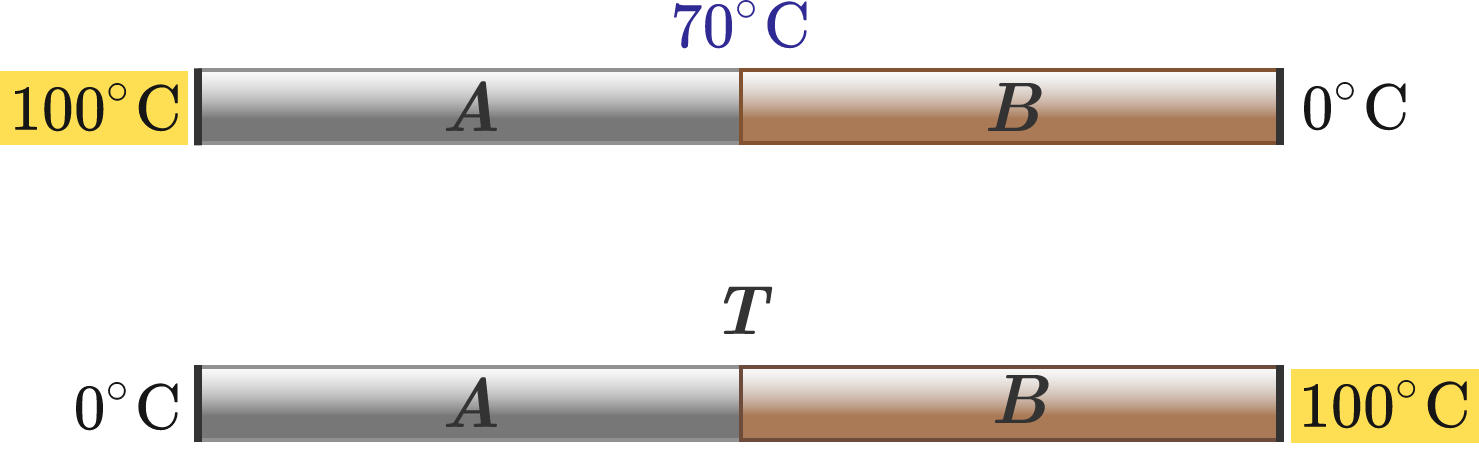Temperatures Interchanged

Ram has joined two rods $A$ and $B$ of different materials but the same length and cross-sectional area end to end, as shown. When he maintained the end of rod $A$ at $100\, ^\circ\text{C}$ and the end of rod $B$ at $0\, ^\circ\text{C},$ the temperature at the junction was found to be $70\, ^\circ\text{C}.$

If he reverses the end temperatures, i.e. if he maintains the end $A$ at $0\, ^\circ\text{C}$ and the end of $B$ at $100\, ^\circ\text{C},$ what will be the junction temperature?Try my World of Physics to solve many problems like this one.

×